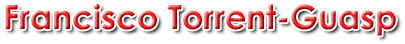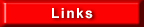F R A N C I S C O  T O R R E N T - G U A S P  -  V E N T R I C U L A R  M Y O C A R D I A L  B A N D  -  N E W  C O N C E P T  O F  T H E  H E A R T  S T R U C T U R E  A N D  F U N C T I O N ...